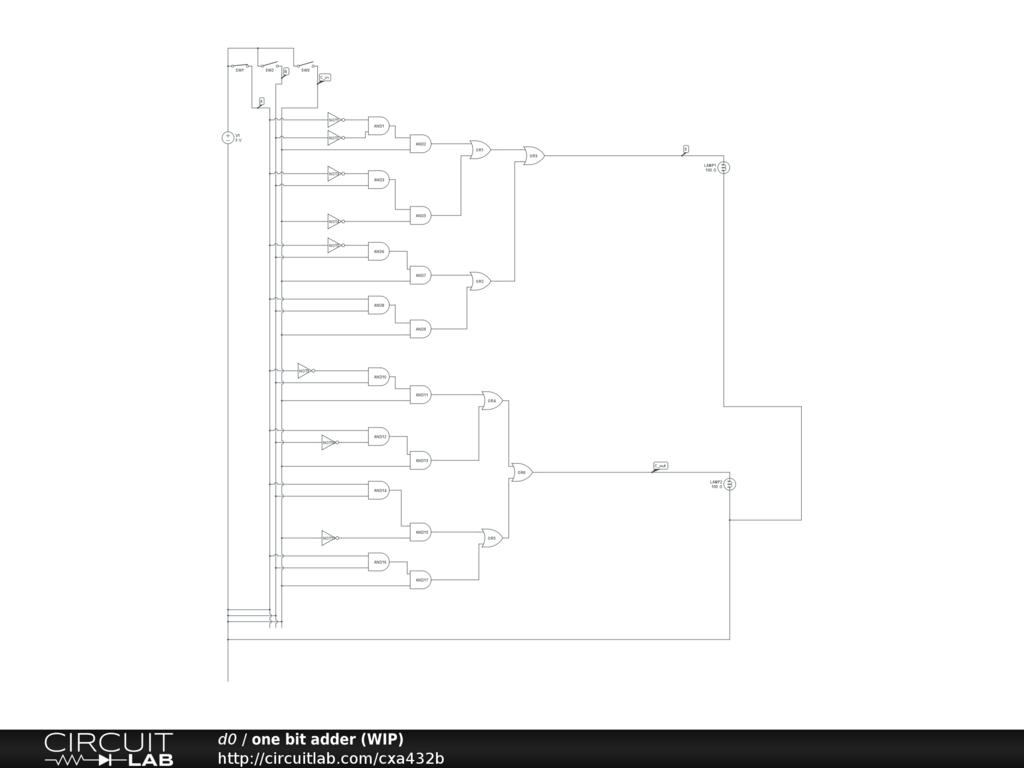## Strange voltage results in my one bit adder

 Hello, everyone! I am working on a very simple project and I have only very basic proficiency regarding electronics. I have a minor problem with my circuit. It delivers strange results. I suspect it is my wiring.Problem description: When I run the DC solver, I get -125.0fV instead of 5V on the output S, and the value on node A is -1.375pV instead of 5V. However, the value of the voltage source is 5V. Also notice that the switch for operand A is closed (so that the first operand becomes 1 - 5V). Further details: - 5V should be 1 (true), 0V should be 0 (false). - Circuit don't needs to be minimized. - Node A (first operand), node B (second operand), C_in, S (for sum), C_out Thanks in advance! Daniel by d0 November 23, 2015 Grammar correction: Circuit doesn't needs to be minimized. by d0 November 23, 2015 @d0, Welcome to CL. There are 3 mistakes in your circuit as published on 151124. i) You have shorted A, B and C to the 5V supply negative end (3 wires at the lower left of the schematic); ii) Your circuit has no ground symbol. You need one at the negative end of the 5V supply. For more, see: https://www.circuitlab.com/docs/the-basics/#ground iii) You cannot use the switches the way you have drawn them. You need to add resistors to ground from the switched (gates) side. Something like 1M (1e6 Ohms) would be OK. Here's why... About using a simulator Neither real world nor most simulator components are ideal. The departures from ideal are often different between the two. If these differences between real and simulated components in a circuit are not clearly understood, this can lead to confusion when the results of a even a simple simulation don’t match those of real world measurements. Therefore, you have to understand exactly what it is you are trying to measure and how the simulation circuit will affect your expected results. Suppose you have a CL switch connected in series with an ideal voltage source set to 1V and you want to measure the Output node of the switch when open and closed. To measure this voltage you use a simple plot expression of V(Output). With the switch closed you’d expect to see V(Output) = 1V. And that’s what you see. OK. With the switch open you’d expect to see V(Output) = 0V. What you actually see is V(Output) = 1V. What’s that all about? Surely the switch must not be simulating properly and is stuck closed? Actually no. The switch and voltage are doing exactly what they should. It’s just that you aren’t expecting these results because you haven’t understood how the simulated circuit differs from a real circuit. No real switch has an infinite OFF state resistance but similarly, there is no such thing as a real open circuit that presents an infinite load impedance. The switches in CL are also not ideal: they have a very large but finite OFF resistance. However, the effective resistance to ground at the Output node using a plot expression, really is infinite. Therefore when operating into an infinite resistance load there would be no difference between the ON and OFF state voltages at the Output node. Note that if a CL Voltmeter element were to be connected from the Output node to ground, then there would be a clear ON/OFF state difference because the CL Voltmeter has a user definable finite resistance with a default value of 1G. When you start using a simulator you have to get into a way of thinking about what the real world really looks like, how it differs from the theoretical world of textbook problems and simple circuit diagrammatic representations and therefore what your measurements are likely to be. A demonstration of the finite off resistance of switches in CL: Note that it is not clearly documented but switches in CL do not have an infinite OFF state resistance. This can lead to unexpected results. For example, a DC plot of this circuit: https://www.circuitlab.com/circuit/586t3d/voltage-controlled-switch-off-state-resistance-01/ with R1 removed from the circuit would show V(output) = 1V. Here's why. The resistance to ground at the Output node is infinite because it is an open circuit. However, the switches in CL are not ideal. They have a very large but finite OFF resistance. Therefore when operating into an open circuit load there would be no difference between the ON and OFF state voltages at the Output node. The example demonstrates this using a DC Sweep probing V(output) with a Decade Sweep of the value of R1 (R1.R) from 1e12 to 1e18 at 10 points/Decade. It can be seen that V(Output) = 0.5V when R1.R is equal to around 1e15 Ohms. This is the undocumented OFF state resistance of the CL voltage controlled switch. by signality November 24, 2015 I should also explain that something else that is not documented in CL (and I have only just noticed this) is that the digital elements appear to have an infinite input impedance: https://www.circuitlab.com/docs/circuit-elements/#digital by signality November 24, 2015 Oh and the gates won't drive a 100R load all the way up to the (internal) 5V supply that the gates assume. :) by signality November 24, 2015